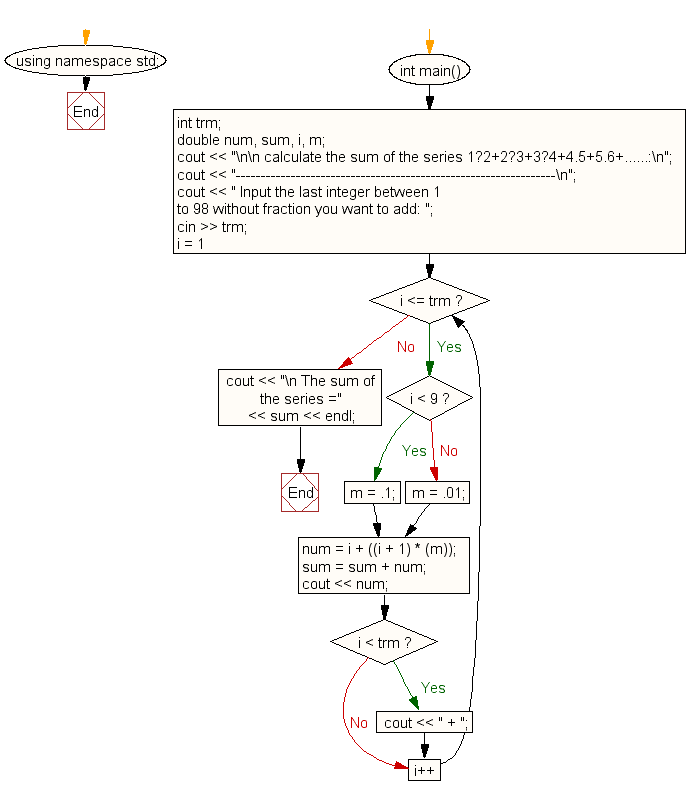﻿ C++ : Calculate the sum of the series 1.2+2.3+3.4+4.5+5.6+…# C++ Exercises: Calculate the sum of the series 1.2+2.3+3.4+4.5+5.6+.....

## C++ For Loop: Exercise-67 with Solution

Write a program in C++ to calculate the sum of the series 1.2+2.3+3.4+4.5+5.6+.......

Sample Solution:-

C++ Code :

``````#include <iostream>
using namespace std;
int main()
{
int trm;
double num, sum, i, m;
cout << "\n\n calculate the sum of the series 1.2+2.3+3.4+4.5+5.6+......:\n";
cout << "----------------------------------------------------------------\n";
cout << " Input the last integer between 1 to 98 without fraction you want to add: ";
cin >> trm;
for (i = 1; i <= trm; i++)
{
if (i < 9)
{
m = .1;
}
else
{
m = .01;
}
num = i + ((i + 1) * (m));
sum = sum + num;
cout << num;
if (i < trm)
{
cout << " + ";
}
}
cout << "\n The sum of the series =" << sum << endl;
}
``````

Sample Output:

``` calculate the sum of the series 1·2+2·3+3·4+4.5+5.6+......:
----------------------------------------------------------------
Input the last integer between 1 to 98 without fraction you want to ad
d: 10
1.2 + 2.3 + 3.4 + 4.5 + 5.6 + 6.7 + 7.8 + 8.9 + 9.1 + 10.11
The sum of the series =59.61
```

Flowchart:C++ Code Editor:

Contribute your code and comments through Disqus.

What is the difficulty level of this exercise?

﻿

## C++ Programming: Tips of the Day

What is a smart pointer and when should I use one?

This answer is rather old, and so describes what was 'good' at the time, which was smart pointers provided by the Boost library. Since C++11, the standard library has provided sufficient smart pointers types, and so you should favour the use of std::unique_ptr, std::shared_ptr and std::weak_ptr.

There was also std::auto_ptr. It was very much like a scoped pointer, except that it also had the "special" dangerous ability to be copied - which also unexpectedly transfers ownership.

It was deprecated in C++11 and removed in C++17, so you shouldn't use it.

```std::auto_ptr<MyObject> p1 (new MyObject());
std::auto_ptr<MyObject> p2 = p1; // Copy and transfer ownership.
// p1 gets set to empty!
p2->DoSomething(); // Works.
p1->DoSomething(); // Oh oh. Hopefully raises some NULL pointer exception.
```

Ref : https://bit.ly/3mc9GHE